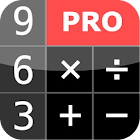PG Calculator (Pro)

All Android applications categories

All Android games categories# PG Calculator (Pro)

27 7.8

7.8 Users
rating

## Screenshots

Description

Do you need a simple calculator which allows you to do basic mathematical operations? Or perhaps you are more demanding user and need to calculate trigonometric functions or logarithms ?

Do you prefer to calculate complete algebraic expressions... or maybe you are a fan of Reverse Polish Notation (RPN) ?

Would you like to have a calculator which looks like 'real' on the screen of your smartphone or tablet?

All of this and much more is offered by PG Calculator Pro - the only calculator you will ever need.

PG Calculator Pro is an application that turns your smartphone or tablet into a powerful scientific calculator with nice and simple user interface.

PG Calculator Pro offers two operational modes: algebraic and RPN:
* In algebraic mode you can enter the entire math expressions such as (2+3)*(4-1) or sin(Pi/3)+cos(Pi/6). It then gives you the correct result according to the conventional mathematical operators.
* In RPN (Reverse Polish Notation) mode you first input your numbers and then tell calculator what to do with them. So for example if you want to calculate (3+4)*5 you simply type as follows: 3 ENTER 4 ENTER + 5 ENTER *. The ENTER key places the number on stack and the operator keys do the task on the numbers

Key features:
* Two operational modes: algebraic and RPN;
* Basic mathematical operations such as addition, subtraction, multiplication and division;
* Exponentiation and the calculation of square roots;
* Basic and inverse trigonometric functions;
* The natural logarithms and the decimal;
* All mathematical operations and scientific functions can work with complex numbers
* You can input numbers in decimal, binary, octal and hexadecimal;
* You can change the appearance of the user interface with built-in 'skins';

There is a library of common mathematical and physical constants which can be used in calculations in both RPN and Algebraic modes. You can also use user-defined variables in your computations.

PG Calculator Pro has built-in Time Value of Money solver and IP Subnets Calculator.

Tags: pg calculator pro apk , pg calculator pro , pg calculator , droid48 pro , polar to rectangular on realcalc , pg calculator scientific apk , application pg calculator pro , pg calculator skins how to change , pg calculator pro 下载

from 27 reviews

"Great"

7.8